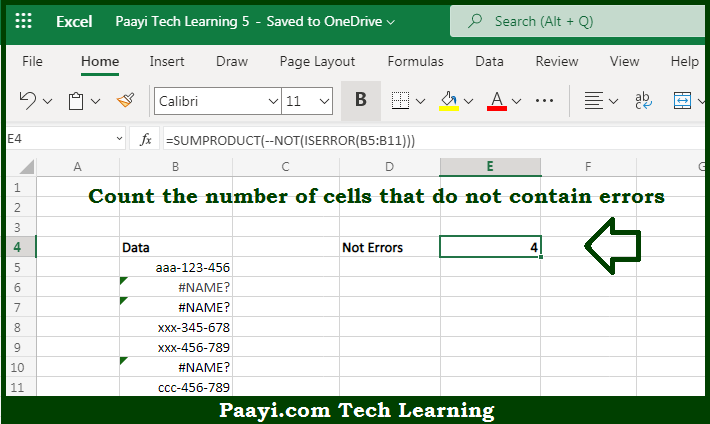# Learn How to Count Cells that Do Not Contain Errors in Microsoft Excel

Written by | 0 Comments | 582 Views

In this article, you will learn how to COUNT various things in Microsoft Excel using a single or combination of functions and its purpose in Microsoft Excel. You will also get to know how to count cells that do not contain errors & see the generic formula

Count Cells that Do Not Contain Errors in Microsoft Excel

The main purpose of this formula is to count the number of cells that do not contain the errors. Here we will learn how to count the range of cells not containing any kind of error in Microsoft Excel.  That implies, with the help of a formula based on the  SUMPRODUCT, ISERROR, and NOT functions you can able to count the number of cells in the range that do not contain any error. So, with the help of this formula, you can able to count the number of cells that do not contain the errors.

General Formula to COUNT Cells that Do Not Contain Errors

=SUMPRODUCT(--NOT(ISERROR(range)))

The Explanation for the COUNT Cells that Do Not Contain ErrorsSo we know that with the help of the given formula above you can able to count the number of cells that do not contain the errors. Here we will learn how to count the range of cells not containing any kind of error in Microsoft Excel. As we know that, in the example provided here, the main purpose is to count the number of cells in a range that does not contain errors. Evaluating from the inside out, we first use the ISERROR function on the entire range. So, with the help of this formula, you can able to count cells that do not contain errors.